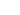# Formula Result

Calculate functions and display a result.

## Options

• Title: Provide a title to the formula result, setting title type and toggle “Title is visible?” to change the visibility on the calculator.
• Add tooltip: Toggle this option if you want to add a tooltip to the result.
• Description: Can add a description under the title. Allow to use markdown markups to format.
• Formula: To calculate the total value. Can use operators (+, -, / , *, ^), numbers (1, 10, 20.5, 1,5) and question and variable values (QA, QB, VA, VB) to build formulas. Besides, you can use IF, AND, OR, and 390 other excel functions. Check out this article to learn more. Decimal numbers without a leading zero will not work (.76)
• Variables: Create variables if you want to use in your formulas.
• Datasheets: Create datasheets to pick up values from a chart to your formula calculations and functions. You can copy and paste the spreadsheets you already have.
• Font Weight: Edit the style of the result (Light, normal or bold). Font Size: Adjust the size of the result.
• Decimals: Set up how many decimals do you want to appear on the result number.
• Prefix: Symbol, number or character do you want to appear before the result number. (e.g. \$)
• Postfix: Symbol, number, words or character do you want to appear after the result number. (e.g. per month)
• Is this questions required?: Toggle if you want to make this number question mandatory required to answer.
• Show/Hide Logic: Toggle this if you want to control if this formula result is shown or hidden under certain circumstances.
• Visibility formula: If the result of the formula is TRUE or a number above 0. The question is visible. If the result is FALSE or 0, it’s hidden.

## Usage in calculations

• Calculate a total value. Can use operators (+, -, / , *, ^), numbers (1, 10, 20.5, 1,5) and question and variable values (QA, QB, VA, VB) to build formulas. Besides, you can use IF, AND, OR, and 390 other excel functions. Check out this article to learn more. Decimal numbers without a leading zero will not work (.76)